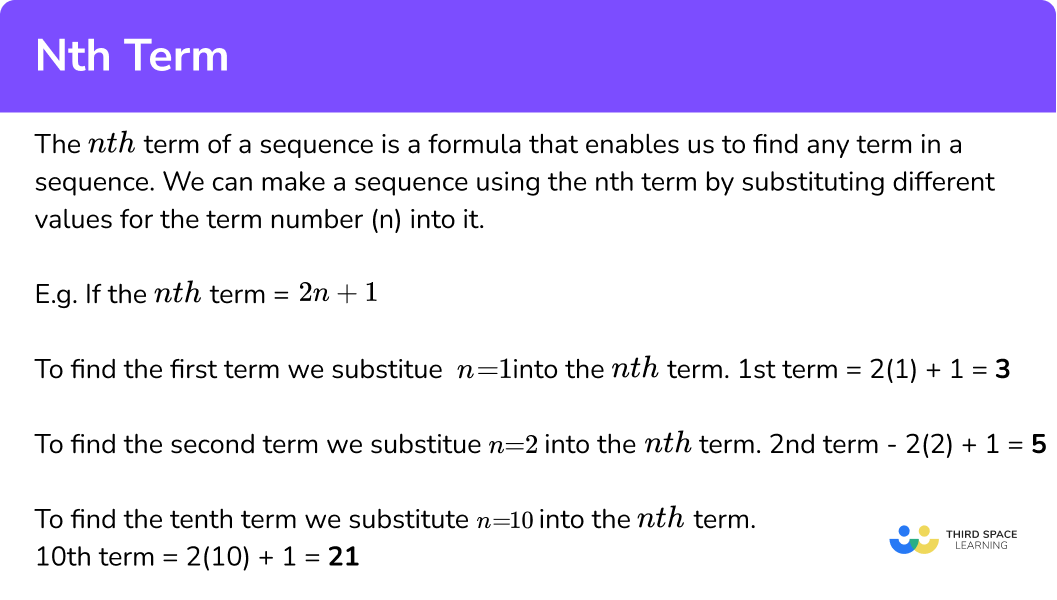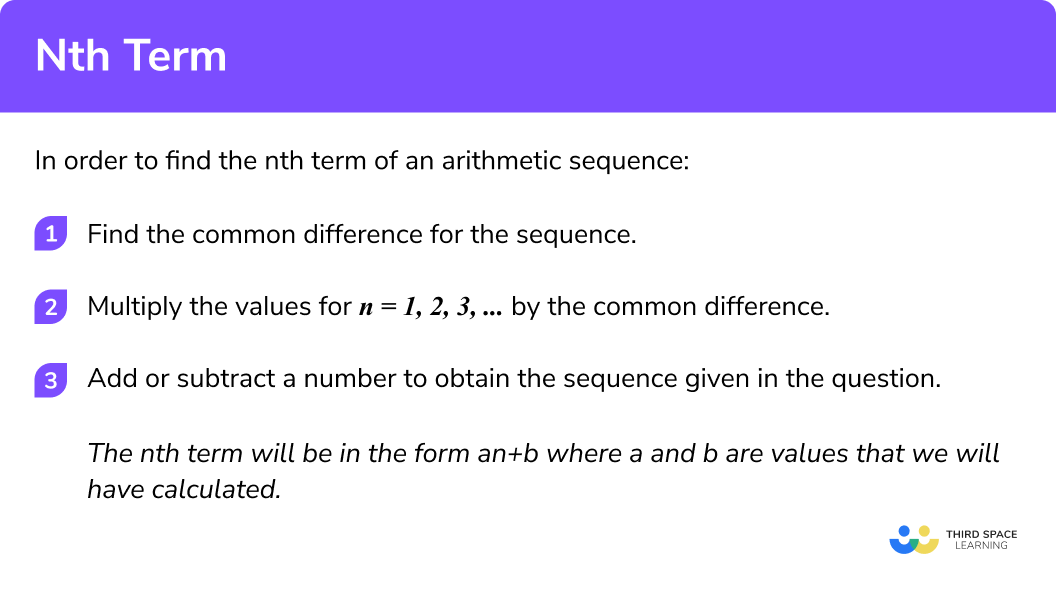GCSE Maths Algebra Sequences

Nth Term Of A Sequence

# Nth Term Of A Sequence

Here we will learn about how to find the nth term of a sequence. You’ll learn what the nth term is and how to work it out from number sequences and patterns.

At the end you’ll find nth term worksheets based on Edexcel, AQA and OCR exam questions, along with further guidance on where to go next if you’re still stuck.

## What is the nth term?

The nth term refers to the position of a term in a sequence. For example the first term has n=1, the second term has n=2, the 10th term has n=10 and so on. The ‘n’ stands for its number in the sequence.

We can make a sequence using the nth term by substituting different values for the term number(n).

To find the 20th term we would follow the formula for the sequence but substitute 20 instead of ‘n‘; to find the 50th term we would substitute 50 instead of n.

For example if the nth term = 2n + 1

• To find the first term we substitute n = 1 into the nth term.

1st term =  2(1) + 1 = 3

• To find the second term we substitute n = 2 into the nth term.

2nd term =  2(2) + 1 = 5

• To find the third term we substitute n = 3 into the nth term.

3rd term =  2(3) + 1 = 7

• To find the tenth term we substitute n = 10 into the nth term.

10th term =  2(10) + 1 = 21

Below are a few examples of different types of sequences and their nth term formula.

Type of Sequence Example nth Term
Arithmetic 6, 2, -2, -6, -10, ...10-4n
Geometric 1, 2, 4, 8, 16, 32, ...2{n-1}
Quadratic3, 9, 19, 33, 51, ... 2n^{2}+1
Cubic2, 22, 78, 188, 370, ...3n^{3}-n

In this lesson, we will look specifically at finding the nth term for an arithmetic or linear sequence.

### What is the nth term?### Related lessons on sequences

Nth term of a sequence is part of our series of lessons to support revision on sequences. You may find it helpful to start with the main sequences lesson for a summary of what to expect, or use the step by step guides below for further detail on individual topics. Other lessons in this series include:

## How to find the nth term

To find the nth term of a sequence use the formula an=a1+(n−1)d.

Here’s how to understand this nth term formula.

The nth term of an arithmetic sequence is given by:

a_{n}=a_{1}+(n-1) d

To find the nth term, first calculate the common difference, d.

Next multiply each term number of the sequence (n = 1, 2, 3, …) by the common difference.

Then add or subtract a number from the new sequence to achieve a copy of the sequence given in the question.

This will give you the nth term term in the form an + b where a and b are unknown values that we will have calculated.

To summarise, in order to find the nth term of an arithmetic sequence:

1. Find the common difference for the sequence.
2. Multiply the values for n = 1, 2, 3.
3. Add or subtract a number to obtain the sequence given in the question.

### Explain how to find the nth term of an arithmetic sequence in 3 steps## nth term formula

The nth term formula for an arithmetic sequence is a_n=a_1+(n-1)d

Where,

a_{n} is the n^{th} term (general term)

a_{n} is the first term

n is the term position

d is the common difference

• The nth term formula for a geometric sequence is:

a_n=a_1(r)^{n-1}

Where,

a_{n} is the n^{th} term (general term)

a_{1} is the first term

n is the term position

r is the common ratio

• The nth term formula for a quadratic sequence is:

an^2+bn+c

Where,

a, b and c are constants (numbers on their own)

n is the term position

a + b + c is the first term

3a + b is the first difference between

2a is the second difference

## nth term examples

### Example 1: find the nth term for an increasing arithmetic sequence

Find the nth term for the sequence 5, 9, 13, 17, 21,

1. Find the common difference for the sequence.

Here, 9 − 5 = 4.

The common difference d = 4.

2  Multiply the values for n = 1, 2, 3, … by the common difference.

Here, we generate the sequence 4n = 4, 8, 12, 16, 20, …. (the 4 times table).

3 Add or subtract a number to obtain the sequence given in the question.

The nth term of this sequence is 4n + 1.

### Example 2: find the nth term for a decreasing sequence, including negative numbers

Find the nth term for the sequence 3, 1, -1, -3, -5, ….

Here, 1 − 3 = -2

The common difference d = -2.

Here, we generate the sequence -2n = -2, -4, -6, -8, -10, … (the multiples of -2).

The nth term of this sequence is -2n + 5  (or 5 − 2n).

### Example 3: find the nth term of a sequence including decimals.

Find the nth term for the sequence 0.2, 0.5, 0.8, 1.1, 1.4, ….

Here, 1.1 − 0.8 = 0.3

The common difference d = 0.3.

Here, we generate the sequence 0.3n = 0.3, 0.6, 0.9, 1.2, 1.5, … (the multiples of 0.3).

The nth term of this sequence is 0.3n − 0.1 or

$\frac{3 n-1}{10}$

### Example 4: find the nth term of a sequence including negatives and decimals

Find the nth term for the sequence -9.1, -8.3, -7.5, -6.7, -5.9, ….

Here, -8.3 − (-9.1) = -8.3 + 9.1 = 0.8

The common difference d = 0.8.

Here, we generate the sequence 0.8n = 0.8, 1.6, 2.4, 3.2, 4, … (the multiples of 0.8).

The nth term of this sequence is 0.8n − 9.9.

### Example 5: find the nth term of a sequence including fractions

Find the nth term for the sequence

$\frac{1}{4}, \frac{5}{8}, 1,1 \frac{3}{8}, 1 \frac{3}{4}, \ldots$

Here,

$\frac{5}{8}-\frac{1}{4}=\frac{5}{8}-\frac{2}{8}=\frac{3}{8}$

The common difference

$d=\frac{3}{8}$

Here, we generate the sequence

$\frac{3 n}{8}=\frac{3}{8}, \frac{3}{4}, 1 \frac{1}{8}, 1 \frac{1}{2}, 1 \frac{7}{7}, \ldots$

$\left ( \text{the multiples of }\frac{3}{8} \right ).$

The nth term of this sequence is

$\frac{3 n}{8}-\frac{1}{8} \text { or } \frac{3 n-1}{8}$

### Example 6: find the nth term when interpreting a pattern

Using the patterns below, write an expression for the number of lines in pattern n.

By counting the number of sides we can see that the first term in the sequence is 12.

The second term in the sequence is 21.

The next term 30.

Here, 21 − 12 = -9

The common difference d = 9.

Here, we generate the sequence 9n = 9, 18, 27, 36, 45, … (the multiples of 9).

The nth term of this sequence is 9n + 3.

### Common misconceptions

• The common difference is used as the constant instead of the multiplier

For example, the sequence 3, 6, 9, 12, 15, … has the nth term 3n but is incorrectly written as n + 3.

• The nth term is incorrectly simplified

For example, if the nth term of a sequence is equal to 6n − 4, the solution would be incorrectly simplified to 2n.

• For decreasing sequences, the nth term has a positive common difference

For example, taking the decreasing sequence -2, -4, -6, -8, -10, … which has the nth term of -2n but is incorrectly stated as 2n which would be an increasing sequence. This is also true with the constant.

### Practice nth term questions

1. Write down the first three terms in the sequence 4n – 7 .

4, -3, -10-3, -10, -174, 11, 18-3, 1, 5\begin{array}{l} 4 \times 1 – 7 = -3\\\\ 4 \times 2 – 7 = 1 \\\\ 4 \times 3 – 7 = 5 \end{array}

2. Below is a table describing the position of each term in an arithmetic sequence and the value of these terms.

State the value of the first term in the sequence.

7.2023.11.5It is an arithmetic sequence meaning the difference between each term is the same.

15.9-8.7 = 7.2 so the difference between each term is 7.2 .

8.7-7.2=1.5 therefore the first term is 1.5 .

3. Below are the first 5 terms of an arithmetic sequence.

Find the n^{th}  term of the sequence.

n+55n-35n+32nThe common difference here is 5 so it is 5n .To get from 5n to our sequence we need to add 3 so our sequence is 5n+3 .

4. Find the n^{th} term formula of the sequence:

10n-20-10n-10-10n-10n+10The common difference  is -10 so it is -10n

We do not need to add or subtract anything here so the nth term is just -10n .

5. The number of petals on a sunflower can be represented as a linear sequence.Write an expression for the number of petals on sunflower n .

2n+52n+32n-35nThe number of petals on the first three flowers are 5, 7 and 9 .

We need to find the n^{th} term of this sequence.

The common difference is 2 so it is 2n .We need to add 3 to the sequence 2n so the expression is 2n+3 .

6. The number of square tiles around a pool generates an arithmetic sequence.How many tiles would there be around a pool of width 30 ?

124120240116Around the first three pools, the number of tiles are 8, 12 and 16 .

The n^{th}  formula for this sequence is 4n+4.

Substituting n = 30 , 4 \times 30 + 4 = 124 .

7. Find the n^{th} term of the linear sequence:

2 \frac{1}{3}, \; \; 2 \frac{2}{3}, \; \; 3, \; \; 3 \frac{1}{3}, \; \; 3 \frac{2}{3}, \ldots

\frac{n}{3}+22n+ \frac{1}{3}3n-22n+3The common difference is \frac{1}{3} so it is  \frac{1}{3} n .

Another way of writing this is \frac{n}{3} .

### nth term GCSE questions

1.  A sequence of patterns is made using triangles.(a) What is the n^{th} term formula for the number of triangles?

(b) How many dark purple triangles would there be in pattern number 100 ?

(3 marks)

(a)

Sequence 1, 3, 5, 7 – common difference is 2

(1)

2n – 1

(1)

(b)

99 (one less than the pattern number)

(1)

2.  (a)  Write down an expression for the n^{th}  term of the following sequence:

-4, -1, 2, 5, 8, ….

(b)  Is the number 101 in this sequence? Show how you decide.

(4 marks)

(a)

Common difference is 3

(1)

3n-7

(1)

(b)

3n  −  7 = 101

(1)

\begin{aligned} 3n&=108\\\\ n&=36 \end{aligned}

Yes it is the 36th term

(1)

3. The n^{th}  of a sequence is 2n + 3 .

The n^{th}  of a different sequence is 5n − 2 .

There are two numbers under 30 that appear in both sequences. What are the two numbers?

(3 marks)

2n + 3: 5, 7, 9, 11, 13, 15, 17, 19, 21, 23, 25, 27, 29,..

(1)

5n − 2: 3, 8, 13, 18, 23, 28, …

(1)

13  and 23

(1)

## Learning checklist

You have now learned how to:

• Recognise arithmetic sequences
• Find the nth term

## Still stuck?

Prepare your KS4 students for maths GCSEs success with Third Space Learning. Weekly online one to one GCSE maths revision lessons delivered by expert maths tutors.

Find out more about our GCSE maths tuition programme.